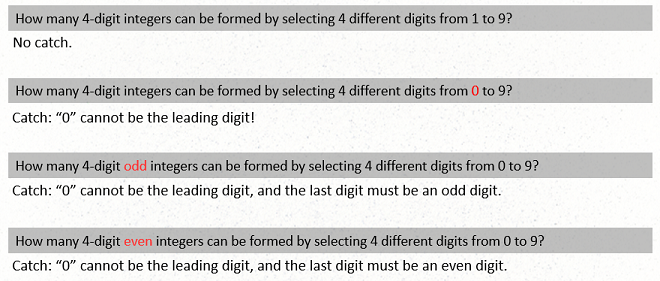Mind the Catches Basic

Video tutorial

Lecture Notes

Problems have catches, that is, they have many traps. Identifying these small nuances and the appropriate techniques to solve them are essential to solving competition mathematics problems.For counting, dependencies are one type of catch. In the last problem, the amount of choices for the leading digit depends on the last digit is a catch you need to account for in your solution. If the last digit is $0$, then there are $9$ choices for the leading digit. If the last digit is $2$, or some other non-zero even number, then there would be $8$ choices for the leading digits.

Tips: choosing a proper solving technique may lead to a neater solution. One way to do this is by carefully designing the order in which the multiplication principles and addition principles are applied. This is illustrated in the first example (# 4771).

Examples

 (4771) How many even $4$- digit integers are there whose digits are distinct?